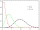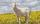# Area of shape - university - math problems

#### Number of problems found: 5

• Poisson distribution - daisiesThe meadow behind FLD was divided into 100 equally large parts. Subsequently, it was found that there were no daisies in ten of these parts. Estimate the total number of daisies in the meadow. Assume that daisies are randomly distributed in the meadow.
• Area and two anglesCalculate the size of all sides and internal angles of a triangle ABC, if it is given by area S = 501.9; and two internal angles α = 15°28' and β = 45°.
• GoatMeadow is a circle with a radius r = 19 m. How long must a rope tie a goat to the pin on the perimeter of the meadow to allow the goat to eat half of the meadow?
• Goat and circlesWhat is the radius of a circle centered on the other circle and the intersection of the two circles is equal to half the area of the first circle? This task is the mathematical expression of the role of agriculture. The farmer has circular land on which g
• SailsWe known heights 220, 165, and 132 of sail. It has a triangular shape. What is the surface of the sail?

We apologize, but in this category are not a lot of examples.
Do you have an exciting math question or word problem that you can't solve? Ask a question or post a math problem, and we can try to solve it.

We will send a solution to your e-mail address. Solved examples are also published here. Please enter the e-mail correctly and check whether you don't have a full mailbox.

Examples of area of plane shapes. Examples for college students.